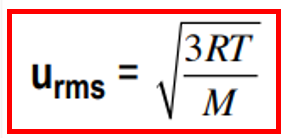# Problem: A gas is at 24°C. To what temperature must it be raised to triple the rms speed of its molecules?

###### FREE Expert Solution

The root mean square equation is shown below:Where: R = 8.314 J/mol-K, T = temperature (K), M= molar mass (kg/mol)

Now if the new rms is 3 times larger than the initial we can write the an equation to represent it.

79% (483 ratings)###### Problem Details

A gas is at 24°C. To what temperature must it be raised to triple the rms speed of its molecules?